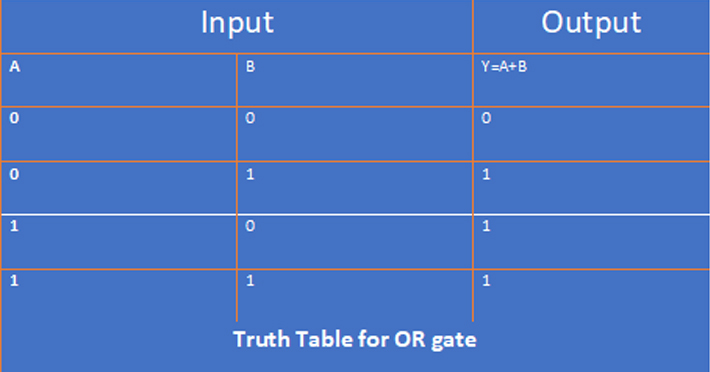# Boolean Truth Table | Truth Table Examples

Published on 01-Oct-2022

## Boolean Truth Table

A table that displays different outputs for different manipulation of variables in a Boolean equation is called a true table.
Truth tables are used to verify the validity of Boolean equations.
A truth table is a kind of mathematical table that is constructed by various arguments of Boolean algebra, Boolean functions, and proportional calculus.  The truth array is used to verify whether the sentence is true for all the values given for a mathematical sentence.

### Truth Table for OR gate:

Suppose there are two input signals A and B for the OR gate and their output signal is Y. Now see the following figure.## Rules:

1.  The equation has to look at how many variables there are.
2.  The table can have multiple inputs, so if n = variable number. The number of rows will be two n numbers.
3.  The value of the last variable is 0 and 1 to fill the column.
4.
And need to create tables by solving the necessary mathematical problems.

## Use of True Tables:

1.  The true table determines the circuit's output, after which the Boolean sum is calculated and the electronics circuit is built.
2. Truth tables are used to prove Boolean theorems.
3. A Boolean sum can be converted to a new sum by simplification.

## Quiz Practice

Correct Answer of previous question :
Total Answred:
Correct:
Wrong:
Accuracy(%):

Tag  #